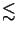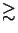Next: 4.1.1 Scalar Diffraction Theory Up: 4. Aerial Image Simulation Previous: 4. Aerial Image Simulation

# 4.1 Principles of Fourier Optics

The foundations of scalar diffraction theory can directly be derived from the most rigorous physical description of light, namely from the Maxwell equations. Applying certain approximations to the general results yields closed-form expressions valid in the optical far field. These approximations combined with a simple model for the phase transformation property of a thin lens establish the Fourier analysis of an imaging system. If the problem is further restricted to Köhler illumination [11, pp. 524-526] and periodic mask patterns, Fast Fourier Transform (FFT) algorithms can be useful for an efficient evaluation of the resulting formulae. Usually the scalar version of Fourier optics is based on the paraxial approximation [111, p. 80], i.e., the angles of the light rays to the optical axis are assumed to be sufficiently small so that small angle approximations can be made. This assumption holds up to numerical apertures of NA0.5 . By not imposing the paraxial approximation scalar models can be applied to NA0.6 . For higher numerical aperture systems polarization effects cannot be neglected and vector diffraction theory is clearly needed to account for the polarization and the inherent coupling between the vector components of the electromagnetic field . For NA0.6 the vector-valued pendant  to the scalar theory of Fourier optics has to be used.Next: 4.1.1 Scalar Diffraction Theory Up: 4. Aerial Image Simulation Previous: 4. Aerial Image Simulation
Heinrich Kirchauer, Institute for Microelectronics, TU Vienna
1998-04-17